## Selina Concise Mathematics Class 10 ICSE Solutions Chapter 23 Graphical Representation (Histograms, Frequency Polygon and Ogives) Ex 23

These Solutions are part of Selina Concise Mathematics Class 10 ICSE Solutions. Here we have given Chapter 23 Graphical Representation (Histograms, Frequency Polygon and Ogives) Ex 23.

Question 1.
Draw histograms for the following distributions :Solution: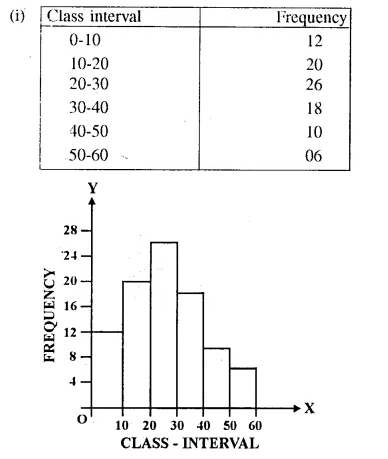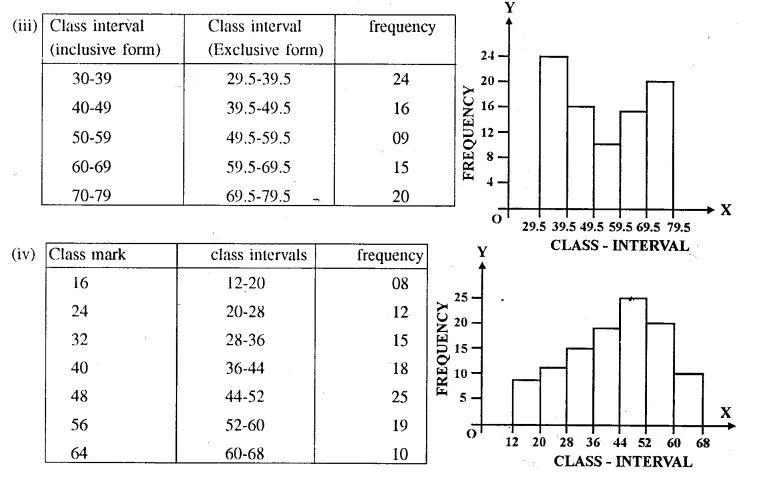Question 2.
Draw a cumulative frequency curve (ogive) for each of the following distributions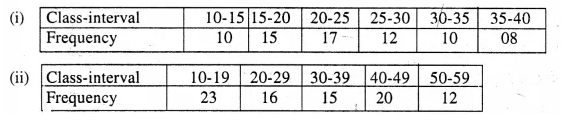Solution: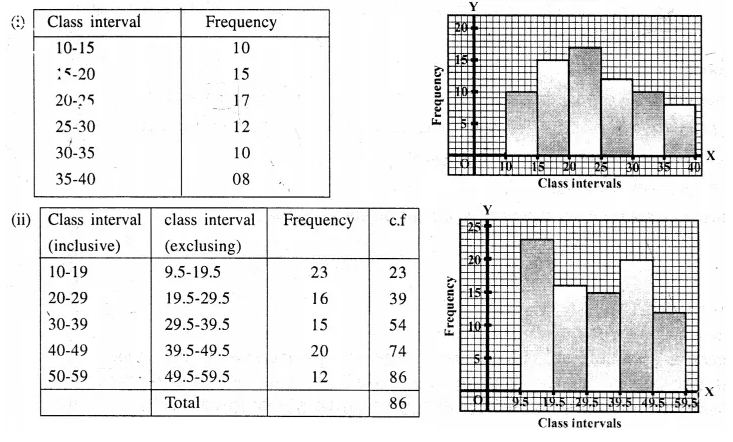Question 3.
Draw an ogive for each of the following distributions :Solution: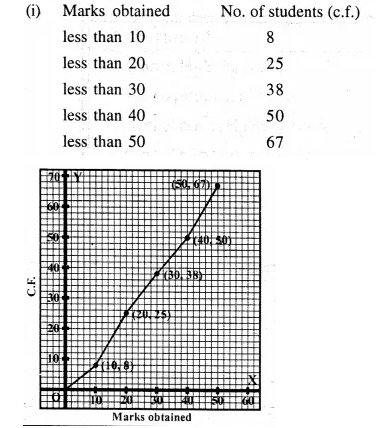Now plotting the points (10, 8), (20, 25), (30, 38), (40, 50) and (50, 67) on the graph and join them with free hand to obtain an ogive as shown in the graph.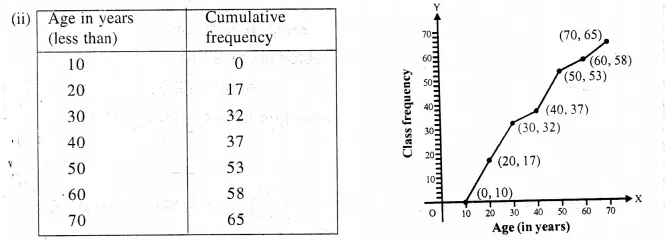Now plot the points (10, 0), (20, 17), (30, 32), (40, 37), (50, 53), (60, 58) and (70, 65) on the graph and join them with free hand to get an ogive as shown in the graph.

Question 4.
Construct a frequency distribution table for the numbers given below, using the class intervals 21-30,31-40…. etc.
75, 67, 57, 50, 26, 33, 44, 58, 67,75, 78, 43, 41, 31, 21, 32, 40, 62, 54, 69, 48, 47,51,38, 39,43,61, 63, 68, 53, 56, 49, 59, 37, 40, 68, 23, 28, 36 and 47.
Use the table obtained to draw:
(i) a histogram
(ii) an ogive
Solution: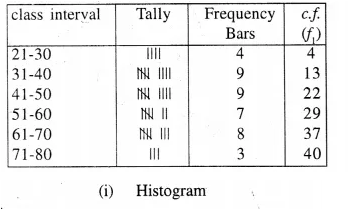(ii) Ogive :
We plot the points (30, 4), (40, 13), (50, 22), (60, 29); (70, 37) and (80, 40) on the graph and join them in free hand to obtain an ogive.

Question 5.
(a) Use the information given in the adjoining histogram to c construct a f frequency table.
(b)Use this table to construct an ogive.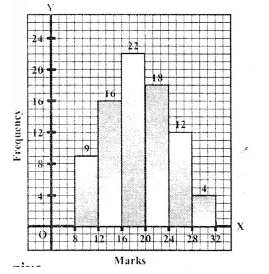Solution:
From the histogram given, the required frequency table will be as given below.Plot the points (12, 9), (16, 25), (20, 47), (24, 65), (28, 77), (32, 81) on the graph and join them with free hand to get an ogive as shown.Question 6.(a) From the distribution given above, construct a frequency table, (b) Use the table obtained in part (a) to draw :
(i) a histogram
(ii) an ogive.
Solution:
Difference in consecutive class marks. = 17.5 – 12.5 = 5
∴ first class interval will be : 10-15 and so on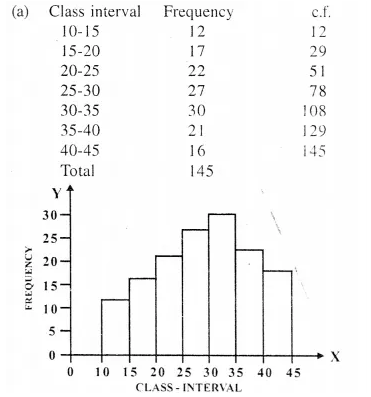(ii) We plot the point (15. 12), (20. 29), (25. 51), (30, 78). (35, 108), (40. 129) and (45, 145) on the graph and join them in free hand to obtain the ogive.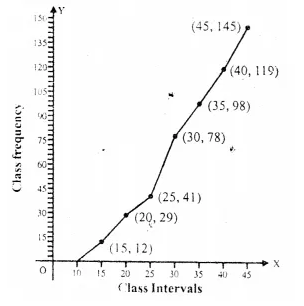Question 7.
Use graph paper for this question.
The table given beiow shows the monthly wages of some factory workers.
(i) Using the table calculate the cumulative frequencies of workers.
(ii) Draw the cumulative frequency curve.
Use 2 cm = Rs. 500, starting the origin at Rs. 6500 on x-axis, and 2 cm = 10 workers on the y-axis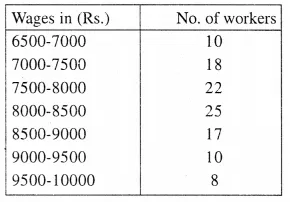Solution: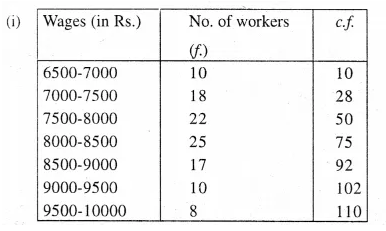(ii) We plot the points (7000, 10), (7500, 28), (8000, 50), (8500, 75), (9000, 92) (9500, 102) and (10000, 110) on the graph and join them in free hand to obtain an ogive.

Question 8.
The following table shows the distribution of the heights of a group of factory workers :(i) Determine the cumulative frequencies.
(ii) Draw the ‘less than’ cumulative frequency curve on a graph paper. Use 2 cm = 5cm height on one axis and 2 cm = 10 workers on the other.
Solution: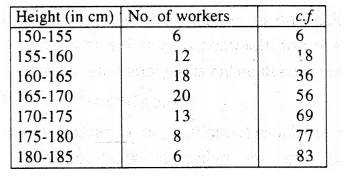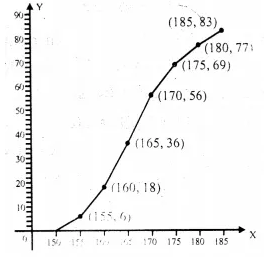We plot points (155, 6), (160, 18), (165, 36),
(170, 56), (175, 69), (180, 77) and (185, 83) on the graph and join them in free hand to obtain an ogive.

Question 9.
Construct a frequency distribution table for each of the following distributions :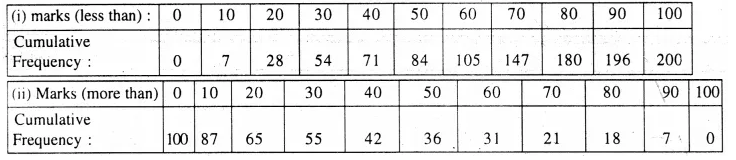Solution: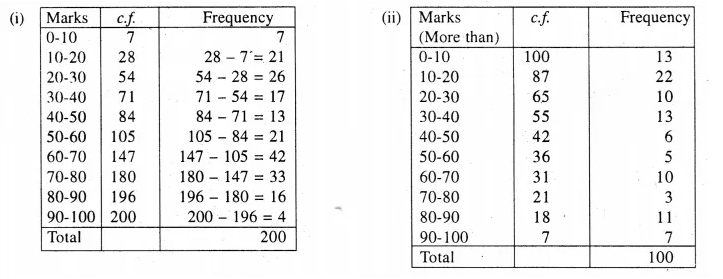Hope given Selina Concise Mathematics Class 10 ICSE Solutions Chapter 23 Graphical Representation Ex 23  are helpful to you.

If you have any doubts, please comment below. We try to provide online math tutoring for you.